# Continuously Compounded Interest

The interest earned on both the initial principal invested and the accumulated interest from previous periods.

Continuously compounding interest is the interest earned on both the initial principal invested and the accumulated interest from previous periods. When interest is said to be constantly compounded, it is compounded at every point in time.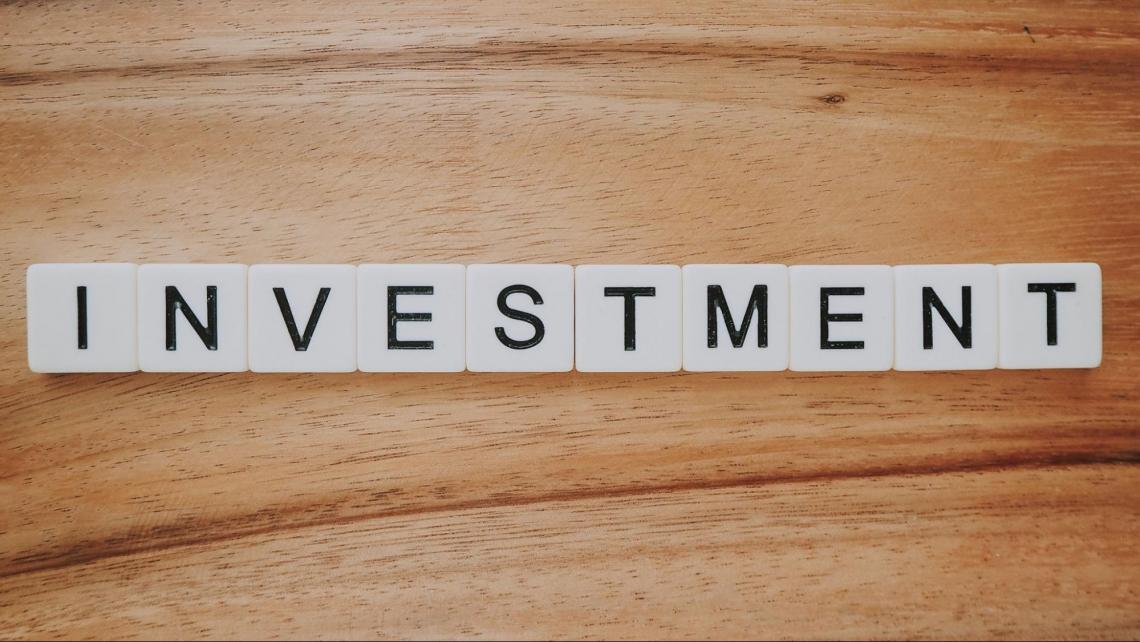Investments usually have interest that can be compounded:

• Annually (once a year),
• Semiannually (twice a year),
• Quarterly (once every three months),
• Monthly (once a month).

Continuous compounding, however, is an extreme case of compound interest as interest is being added and reinvested momentarily instead of at specific and distinct points in time. That is why it is also known as instantaneous compounding.

In reality, this idea is impossible to implement, but it is used in the finance industry as a benchmark for the most extreme case of compounding interest.

One may think that money that is compounded continuously yields an infinite sum of money. However, a formula calculates the future value of a principal whose interest is being compounded instantaneously.

At the same growth rates, it was continuously compounding interest yields the most considerable future value of an asset compared to interest compounded yearly, semiannually, quarterly, monthly, daily, etc. That is because the asset will always earn and reinvest interest.

## Overview of compound interest

Compound interest is the interest earned on the principal amount and the interest piled up from the earlier periods. It is also considered the interest on the previously accrued interest.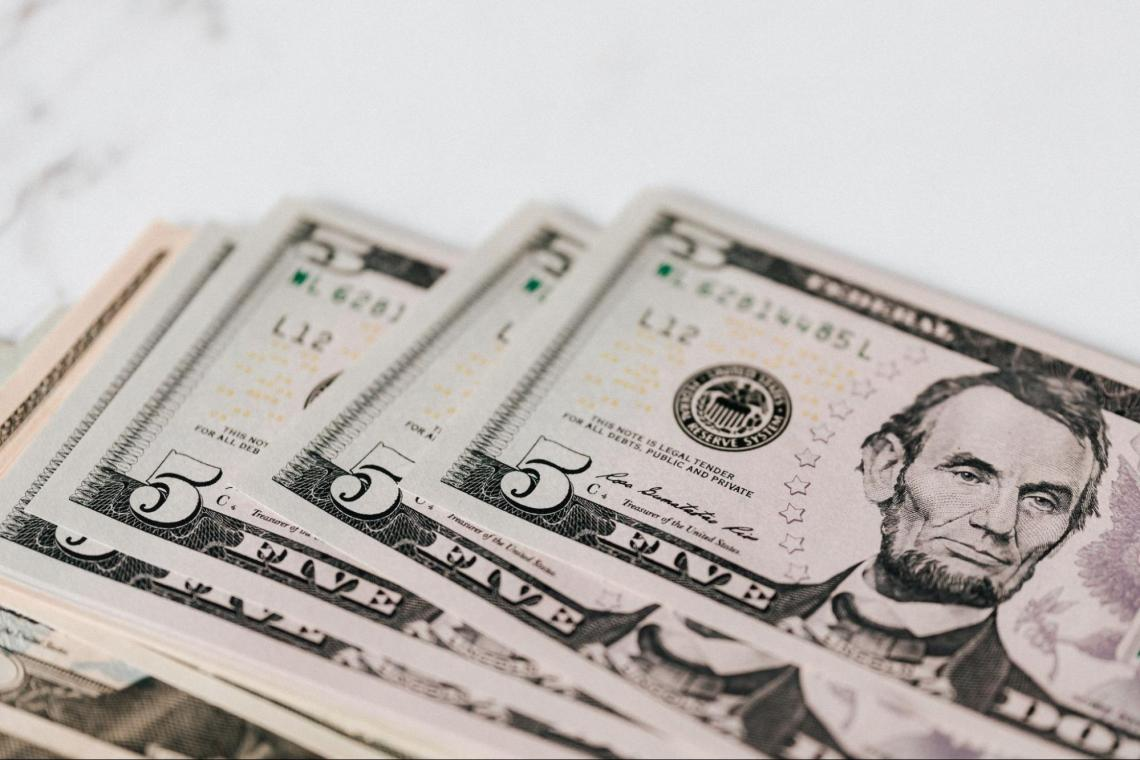An account that compounds the interest in addition to the principal will have a larger future balance than an account that only rewards interest on the principal deposit. That is because the former performance will earn interest on the accumulated interest, unlike the latter.

The interest-only dedicated to the principal amount is called simple interest. It is the opposing idea to compound interest, in which the interest earned also includes the previously piled-up interest, in addition to the principal

Let us see how accounts with compound interest earn more money than accounts with simple interest with an example. Assume there are two accounts you can deposit \$1,000 in:

1. An account that pays 10% simple interest (no interest on interest) means that each year, \$1,000 * 10% = \$100 will be added to the account.
2. An account that pays 10% interest compounded yearly (includes interest on interest).

What would the balance of those accounts be one year after depositing \$1,000?

• The first account will have a balance of: 1,000 + \$100 = \$1,100.
• The second account will also have a balance of: \$1,000 * (1 + 0.1) = \$1,100.Note that both accounts will have the same balance after one year. That is because there is no interest from previous periods to be compounded so that both accounts will have the same balance at the end of the first year.

What would the balance of those accounts be two years after depositing \$1,000?

• The first account will have a balance of: \$1,000 + \$100 + \$100 = \$1,200.
• The second account will have a balance of: \$1,100 * (1 + 0.1) = \$1,210.

Notice that starting from the end of year 2, the second account (the one with compound interest) will have a larger balance than the first account (the one with simple interest). Also, the difference between the balances (currently \$10) will widen year by year.

What would the balance of those accounts be three years after depositing \$1,000?

• The first account will have a balance of: \$1,000 + \$100 + \$100 + \$100 = \$1,300.
• The second account will have a balance of: \$1,210 * (1 + 0.1) = \$1,331.

Note that at the end of the third year, the gap between the two accounts has widened from \$10 to \$31.

## Annual, semiannual, quarterly, and monthly compounding

In the previous example, the interest rates are quoted as annual, meaning that interest was earned at the end of each year. Nevertheless, interest rates can also be cited as semiannual, quarterly, and monthly.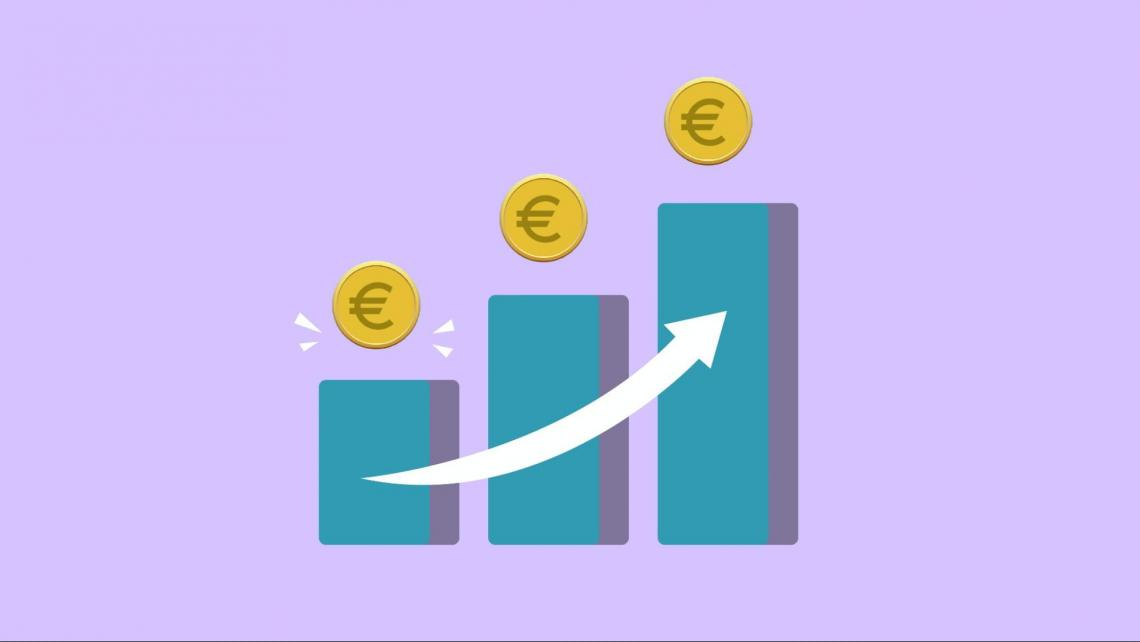Differently quoted interest rates have interest compounded at different rates:

• Semiannual compounding compounds the interest twice per year (once every six months),
• Quarterly compounding compounds the interest four times a year (once every three months),
• Monthly compounding compounds the interest 12 times a year (once a month)

The more frequently the interest is compounded, the more compound interest will be accumulated. That is to say, with same-sized deposits and equal interest rates, the account that monthly compounding will have higher future value than the accounts with annual compounding.

If an account rewards an interest rate of 10% compounded semiannually, the principal earns a 5% interest every six months. However, if the interest were compounded quarterly, the principal would earn 2.5% interest every three months.The formula for calculating the future value of a principal with multiple rounds of compounding in a year is:

FV = PV [1 + i/n]^nt

• FV is the value of the account or the asset at a later date,
• PV is the current balance of the account or the current value of the asset,
• i is the interest rate or rate of growth,
• n is the number of times the interest is being compounded in a year (hence n=2 for semiannual compounding, n=4 for quarterly compounding, and n=12 for monthly compounding),
• t is the number of years after which we want to calculate the future value of the account or asset.

With an example, let us see how accounts with more frequent compounding interest (larger n) earn more money than accounts with less frequent compounding interest (smaller n).

Assume there are several accounts you can deposit \$1,000 at an interest rate of 12%:

1. The first account compounds interest annually (n=1),
2. The second account compounds interest semiannually (n=2),
3. The third account compounds interest quarterly (n=4),
4. The fourth account compounds interest monthly (n =12).

The following table shows the future values of the four accounts after five years (t=5):

Future Value after 5 years
AccountFormulaFuture Value After 5 Years

First Account

(Annual)

FV = \$1,000 [1 + 0.12]5\$1,762.34

Second Account

(Semi-annual)

FV = \$1,000 [1 + 0.122]2*5\$1,790.48

Third Account

(Quarterly)

FV = \$1,000 [1 + 0.124]4*5\$1,806.11

Fourth Account

(Monthly)

FV = \$1,000 [1 + 0.1212]12*5\$1,816.70

According to the table, the fourth account with monthly compounding interest has the highest FV after five years showing that accounts with more frequent compounding will generate more money compared to other accounts or assets with less frequent compounding.

Excel has specific functions that can automatically calculate these values with ease.

## Understanding continuous compounding

As mentioned, interest can be compounded more than once a year. It can be compounded semiannually, quarterly, monthly, and so on. However, interest can also be theoretically compounded continuously (that is to say, at every instant).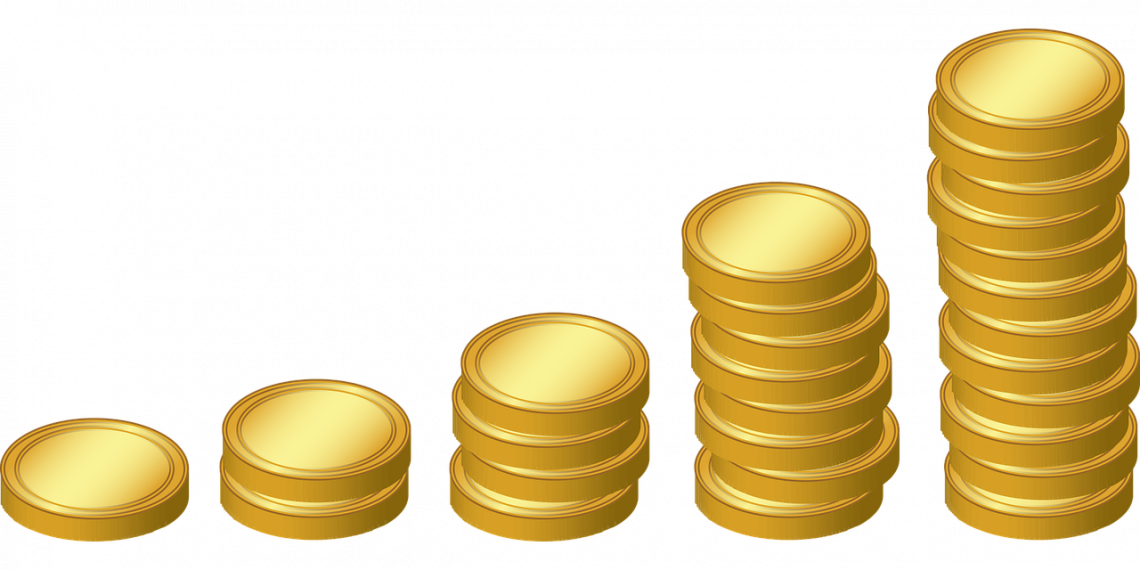Continuous compounding differs from yearly, semiannual, and other compounding forms since interest isn't compounded discreetly, meaning that there is no particular instance that the interest gets compounded. Instead, interest is constantly compounding: at every moment in time.

With a little extra effort, we can also realize that this type of compounding yields the highest return possible on any asset or bank account compared to other discrete forms of compounding interest.

When interest gets compounded instantaneously, you are theoretically making the most amount of compound interest possible. That is because we split our timeline into infinitely small periods, and interest accrues with each passing period.

Although it seems that continuous compounding can yield an infinite amount of compounded interest, that is not the case. There is a mathematical way of calculating the future value of an asset or account characterized by instantaneous compounding.

## Deriving the continuously compounding interest formula

The formula for the future value of an asset or account with continuous compounding can be derived from the formula of the future value of a principal with multiple rounds of compounding in a year mentioned earlier:

FV = PV [1 + i/n]^nt

When interest was compounded monthly, we replaced n by 12. When it was quarterly, we replaced n with 4. In the case of continuous compounding, n is an infinitely large number.

Since we can't replace n because it would be mathematically impossible to calculate FV, we will have to work with limits. That is to say, n → + (tends to infinity) instead of n=+ (equals infinity).

Hence, we can start calculating the future value of an account with instantaneous compounding as follows.

FV = lim (n→+infinity) PV [1 + i/n]^nt = PVe^it

Where e is a mathematical constant known as Euler's number, where e ≈ 2.718282.

How did Euler's number make its way into the equation? It is because of one of the mathematical formulas concerning limits, which states:

lim (n→+infinity) (1+ a/x)^x = e^a

Applying this formula to the FV for continuous compounding, we will have:

lim (n→+infinity) (1+ i/n)^n = e^i

Therefore, the formula for the FV with instantaneous compounding is:

FV = PVe^it

## Example of an account characterized by continuous compounding

Suppose you can invest \$1,000 in an account for five years, which yields an interest rate of 12% compounded continuously. We can calculate the future value of this account balance at the end of the fifth year by using the formula.

FV = PVe^it = \$1,000 * 2.7182820.12*5 = \$1,822.12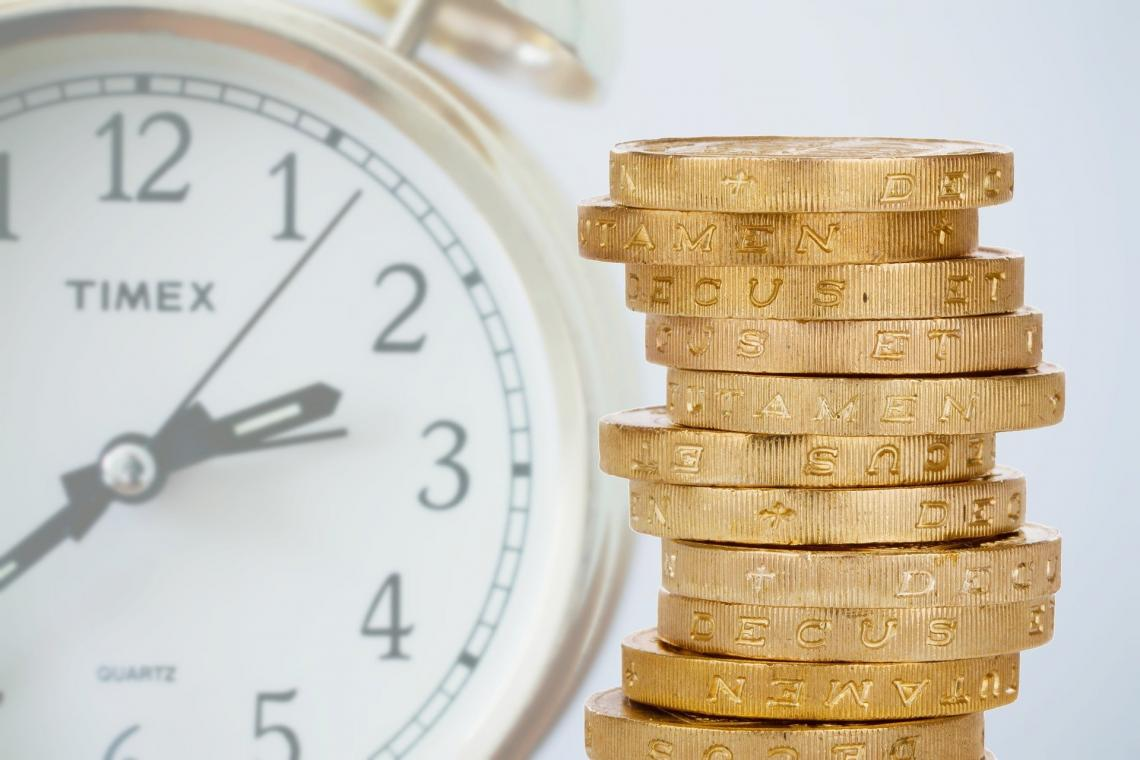With the same parameters (same i and same t), the other accounts worked with previously had lower future values. Therefore, accounts with continuous compounding offer the highest return on investment compared to other accounts which offer discrete compounding.

The following table provides a summary of returns of five accounts with different ways of compounding interest, along with their annualized ROIs (or effective annual rates).

Future Value after 5 years and Effective Annual Rates
AccountFormulaFuture Value After 5 YearsEffective Annual Rates

First Account

(Annual)

FV = \$1,000 [1 + 0.12]5\$1,762.3412%

Second Account

(Semi-annual)

FV = \$1,000 [1 + 0.122]2*5\$1,790.4812.36%

Third Account

(Quarterly)

FV = \$1,000 [1 + 0.124]4*5\$1,806.1112.55%

Fourth Account

(Monthly)

FV = \$1,000 [1 + 0.1212]12*5\$1,816.7012.68%

Fifth Account

(Continuous)

FV = \$1,000 * 2.7182820.12*5\$1,822.1212.75%

As mentioned, the account with continuous compounding had the highest FV after five years. No other account with the same interest rate (12%) can have a higher FV after five years than the account with instantaneous compounding.

In addition, I noticed that effective annual rates of return for all accounts are greater than 12% (except for the one with yearly compounding). This is always the case with frequent compounding because it factors in the effect of compounding interest.

The account with the highest effective annual rate is the one with continuous compounding, with an annualized ROI of 12.75%; because the interest is compounded continuously has increased the returns on the initial principal so that the effective rate has surpassed 12%.### Everything You Need To Master Excel Modeling

To Help You Thrive in the Most Prestigious Jobs on Wall Street.

Researched and Authored by Vatche Tchelderian | LinkedIn